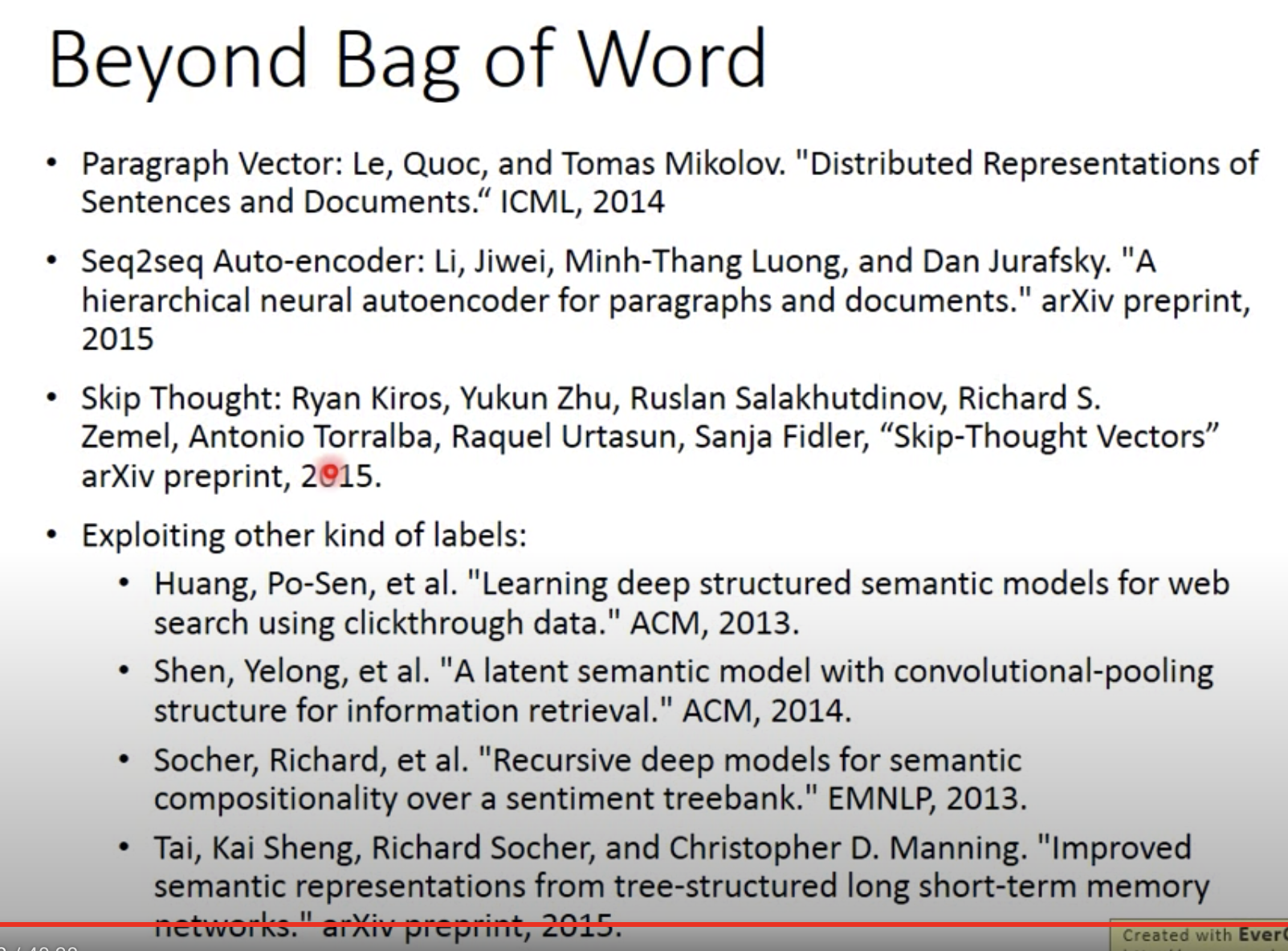# 「机器学习-李宏毅」:Unsupervised Learning：Word Embedding

Word Embedding可以很好的解决这个问题。

Word Embedding有count based和prediction based两种方法。文章主要介绍了prediction based的方法，包括如何predict the word vector? 为什么这样的模型works？介绍了prediction based的变体；详细阐述了该模型中sharing parameters的做法和其必要性。

# Word to Vector

## 1-of-N Encoding

Vector的维度是单词总数，每一维度都代表一个单词。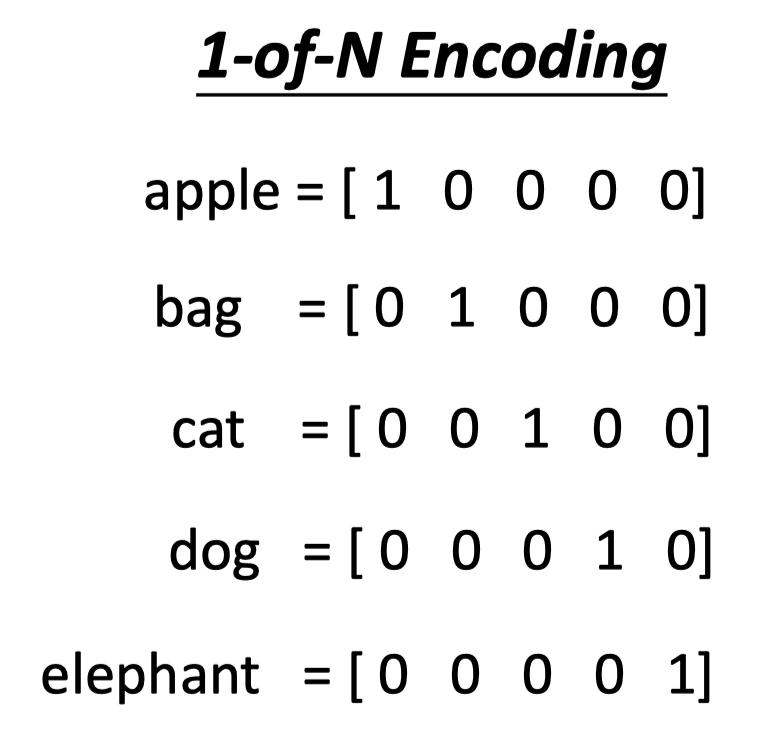1-of-N Encoding的方法简单，但这种向量的表示方式not imformative，即向量表示不能体现单词之间的语义关系。

## Word Class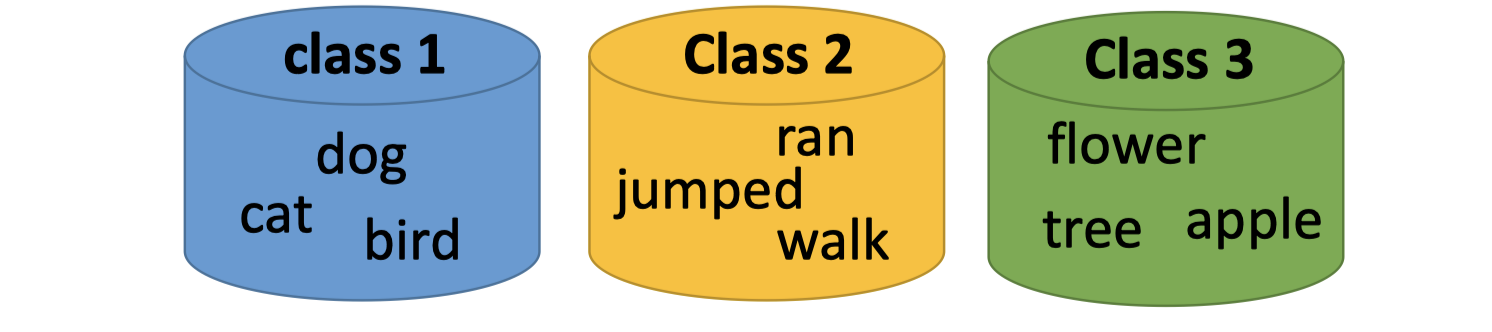## Word Embedding

Word Embedding: Machine learns the meaning of words from reading a lot of documents without supervision.

Word Embedding，机器通过阅读大量文章学习单词的含义，用vector的形式表示单词的语义。训练时只需要给机器大量文章，不需要label，因此是无监督学习。

# Word Embedding

## auto-encoder？

word2vec时，把model中的某一hidden layer的输出作为该单词的向量表示。

## Exploit the Context

A word can be understood by its context.

• Count based

如果两个单词 $w_i$ 和 $w_j$ 在文章中经常同时出现，那么 $V(w_i)$ ( $w_i$ 的向量表示)和 $V(w_j)$ 的向量表示会很close.

E.g. Glove Vector: https://nlp.stanford.edu/projects/glove/

GloVe的表示法有两个亮点：

1. Nearest neighbors：vectors之间的欧几里得距离（或者余弦相似度）能较好表示words之间的语义相似度。

2. Linear substructures：用GloVe方法表示的vectors有有趣的线性子结构。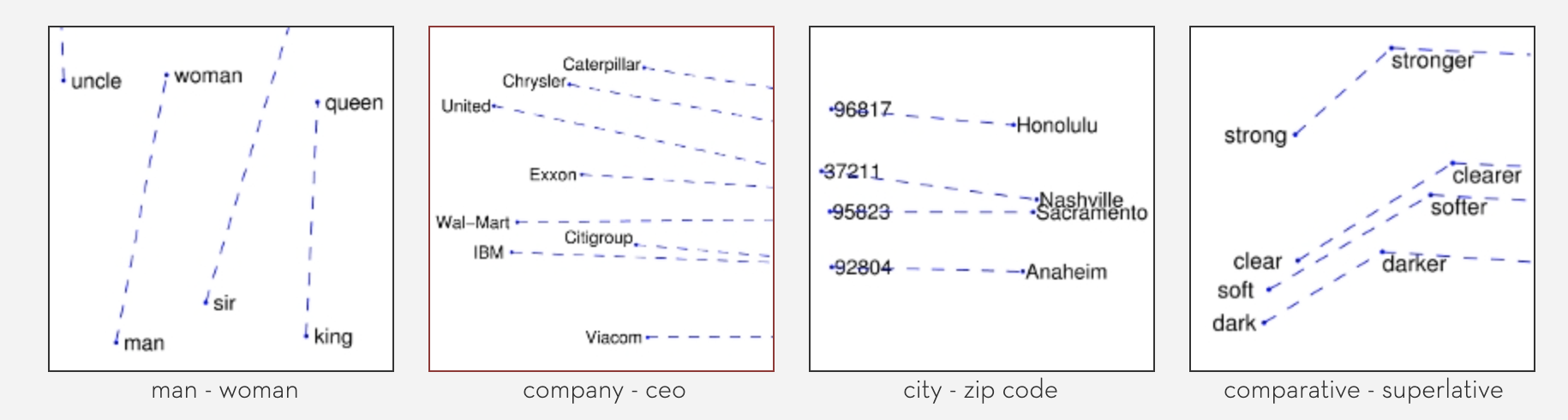• Prediction based

使用预测的方式来表示。

## Prediction based

### How to predict？

prediction based的方法是用前一个单词来预测当前单词。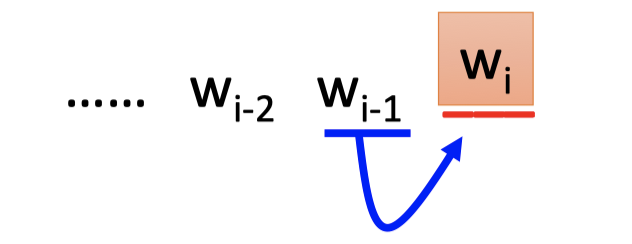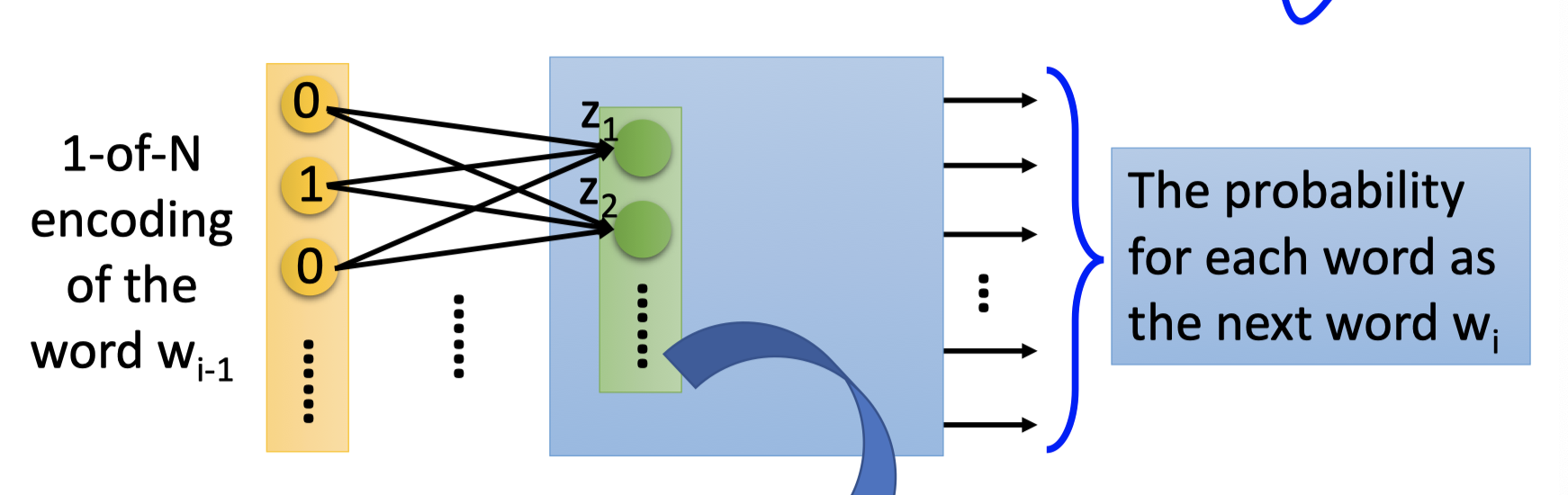NN如上图，$w_{i-1}$ 的1-of-N encoding编码作为输入，输出的vector表示下一个单词是 $w_i$ 的概率。

word2vec : $w_{i-1}$ 的1-of-N encoding编码作为NN的输入，$w_i$ 的向量表示为第一个hidden layer的neurons的输入 $z$ 。

### Why it works?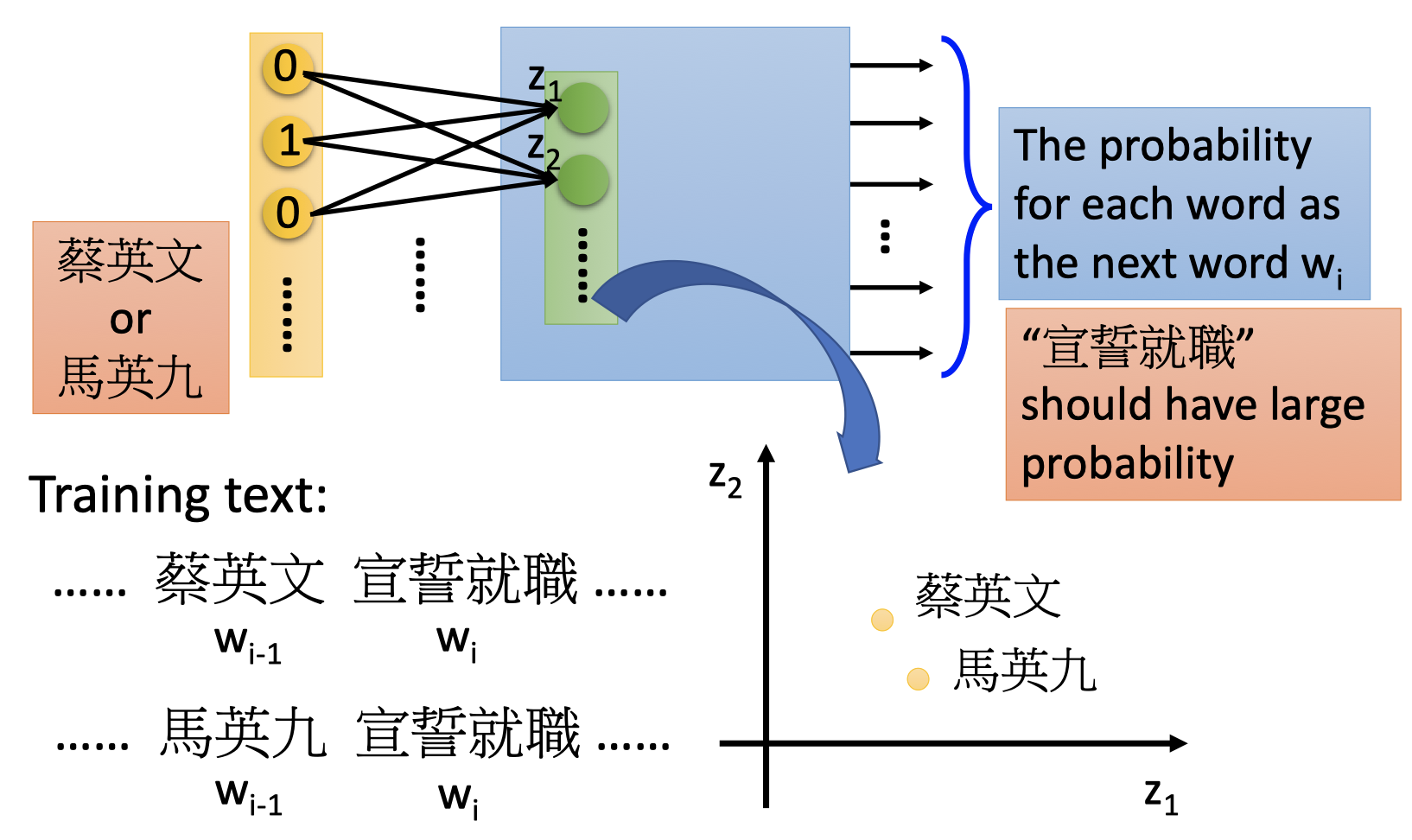### Prediction-based ：Various Architecture

• Continuous bag of word (CBOW) model: predicting the word given its context.

使用单词的前后文（前一个单词和后一个单词）来预测当前单词。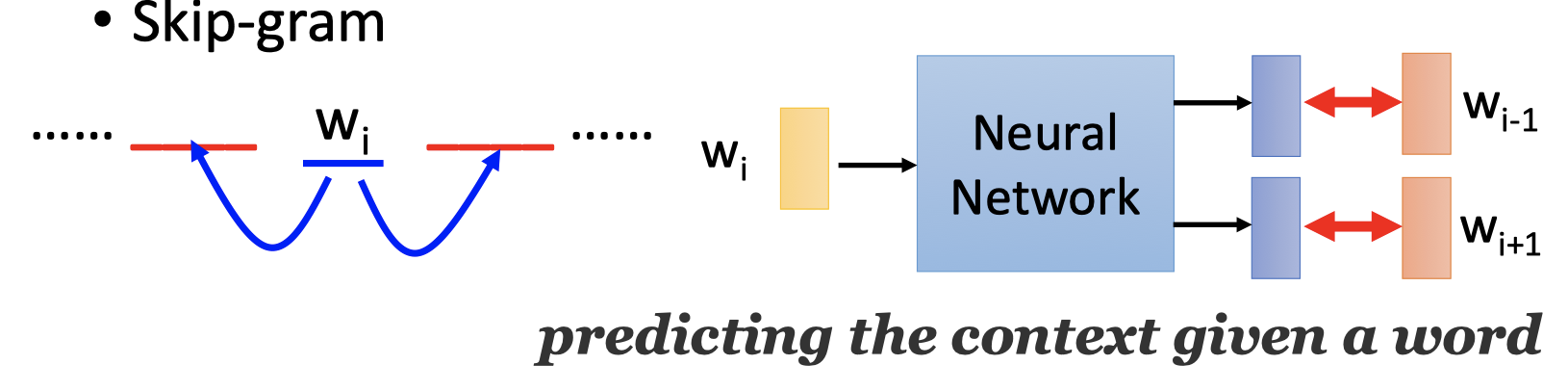• Skip-gram: predicting the context given a word.

使用中间单词来预测单词的前一个单词和后一个单词。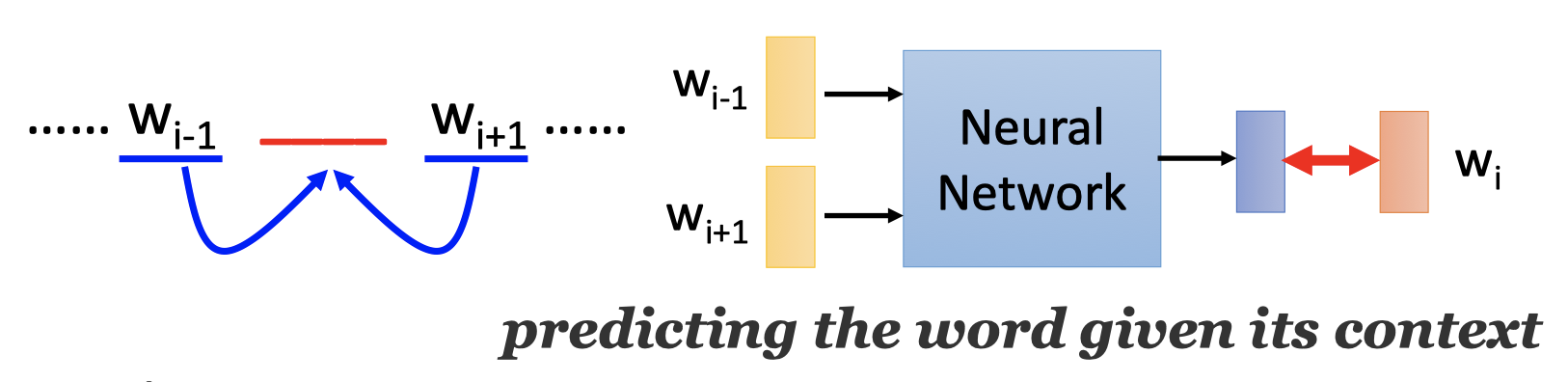### Sharing Parameters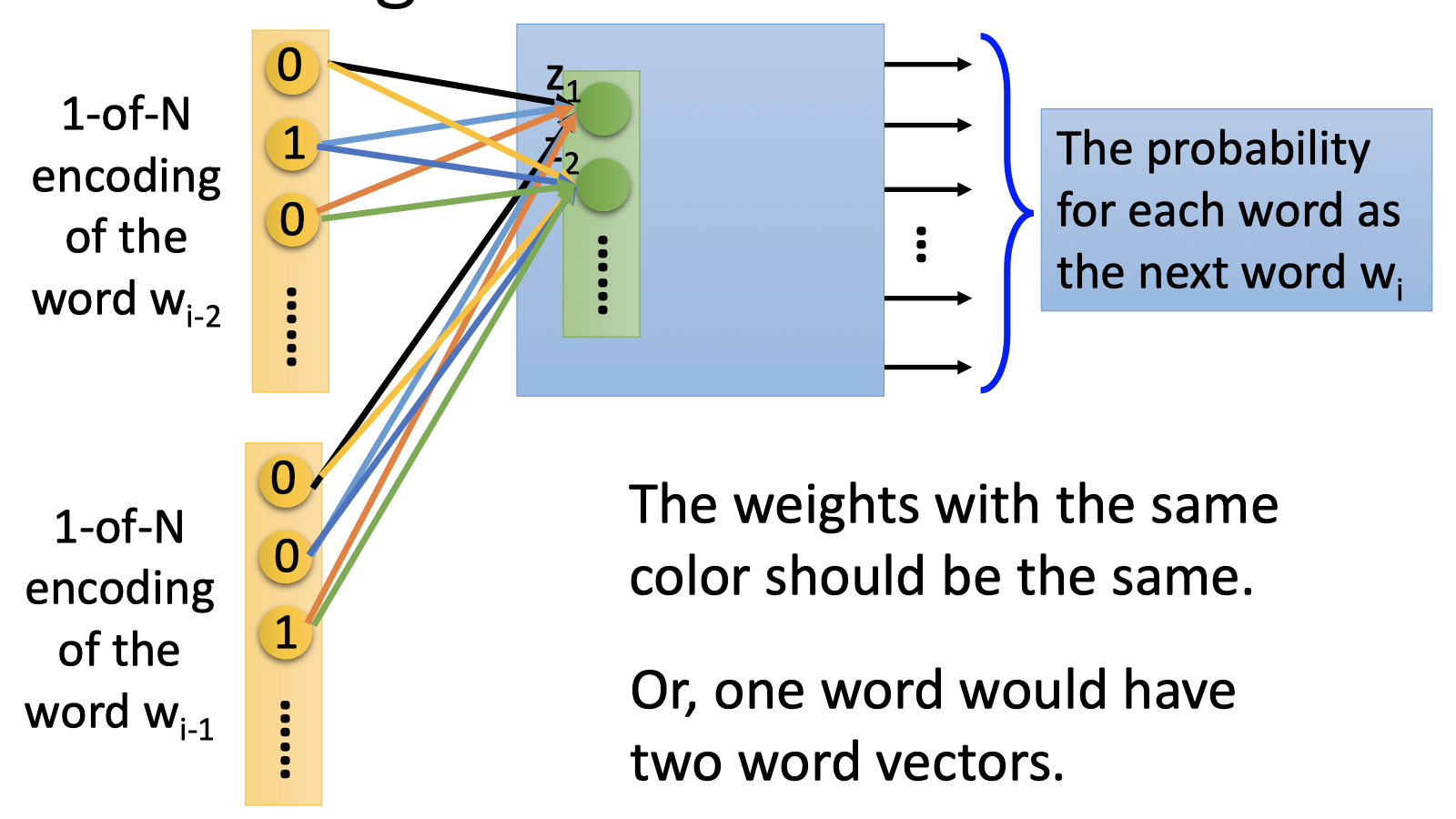How to train sharing parameters?

1. Given the same initialization.(相同的初始化)

2. 原来的参数更新：
$$w_i \longleftarrow w_i - \frac{\partial C}{\partial w_i} \ w_j \longleftarrow w_j - \frac{\partial C}{\partial w_j}$$
虽然有相同的初始化，但在Backpropagation求偏微分时，$\frac{\partial C}{\partial w_i}$ 和 $\frac{\partial C}{\partial w_j}$ 不一样，那么参数 $w_i$ 和 $w_j$ 更新一次后就不同了。

在训练sharing parameters的参数更新：
$$w_i \longleftarrow w_i - \frac{\partial C}{\partial w_i} -\frac{\partial C}{\partial w_j}\ w_j \longleftarrow w_j - \frac{\partial C}{\partial w_j}-\frac{\partial C}{\partial w_i}$$
这样更新后，$w_i$ 和 $w_j$ 仍保持一致。如果有多个单词，亦然。

Word2Vec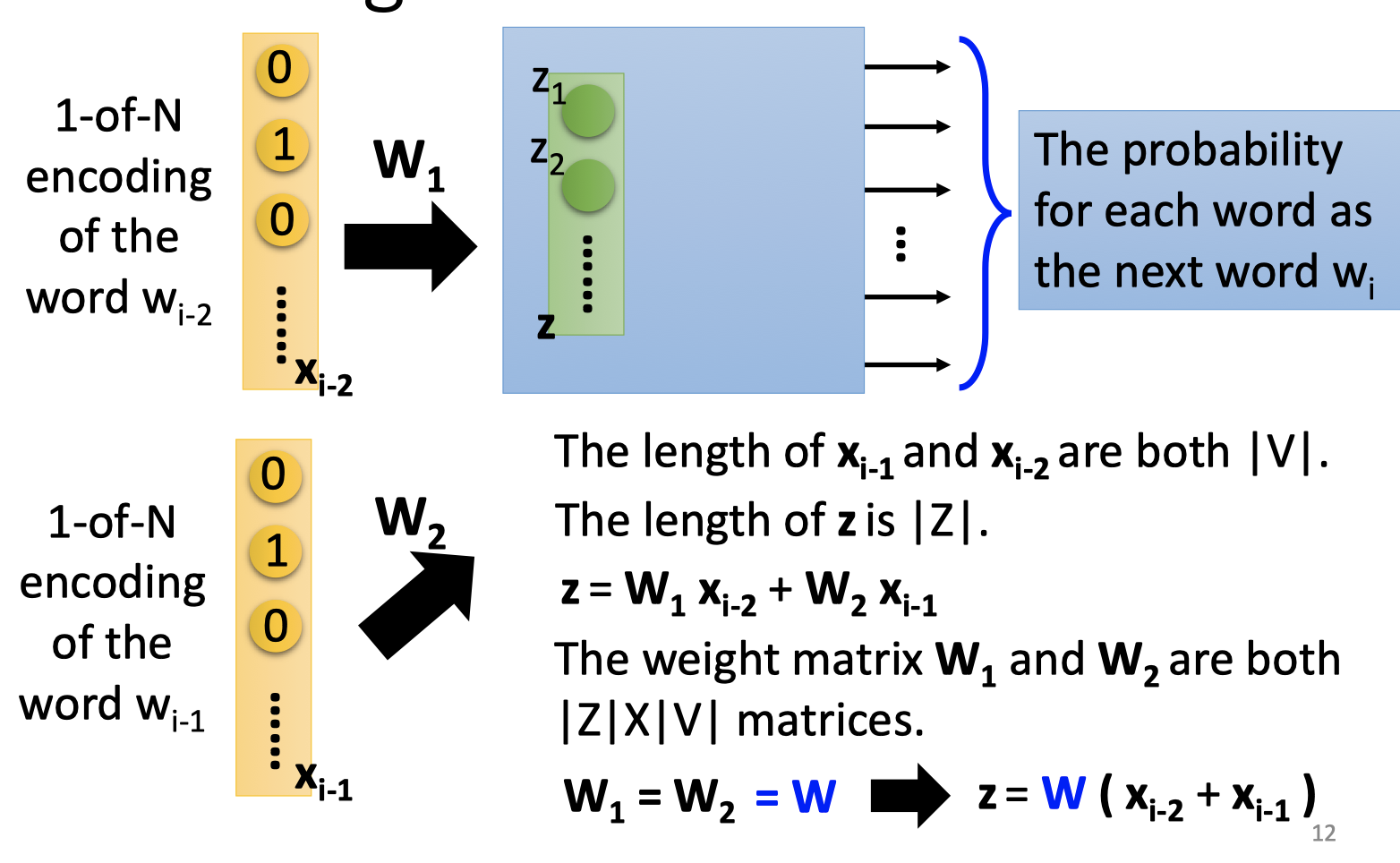Word Embedding能得到一些有趣的特性。

• 向量之间有趣的线性子结构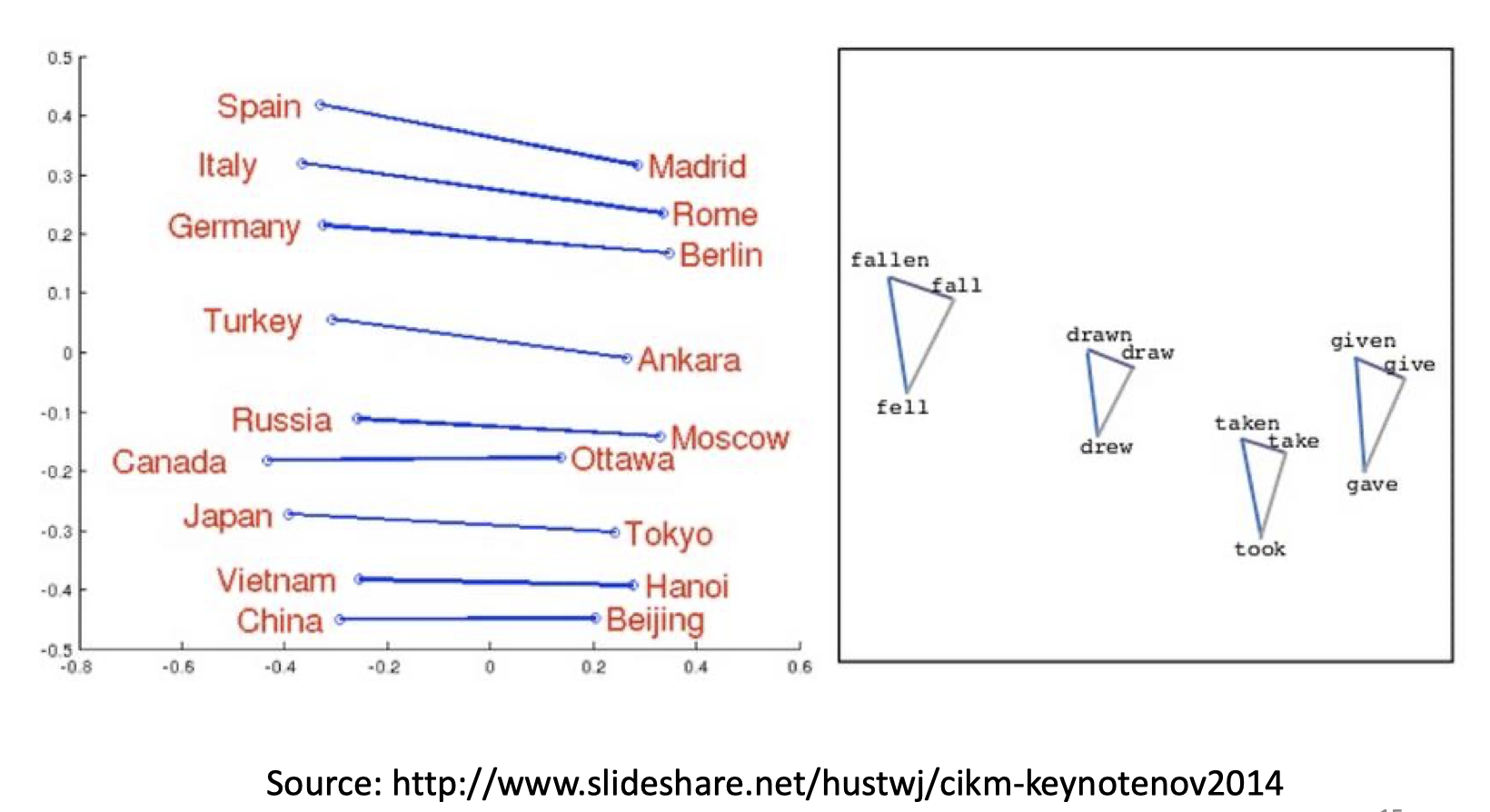• 相近的向量有相近的语义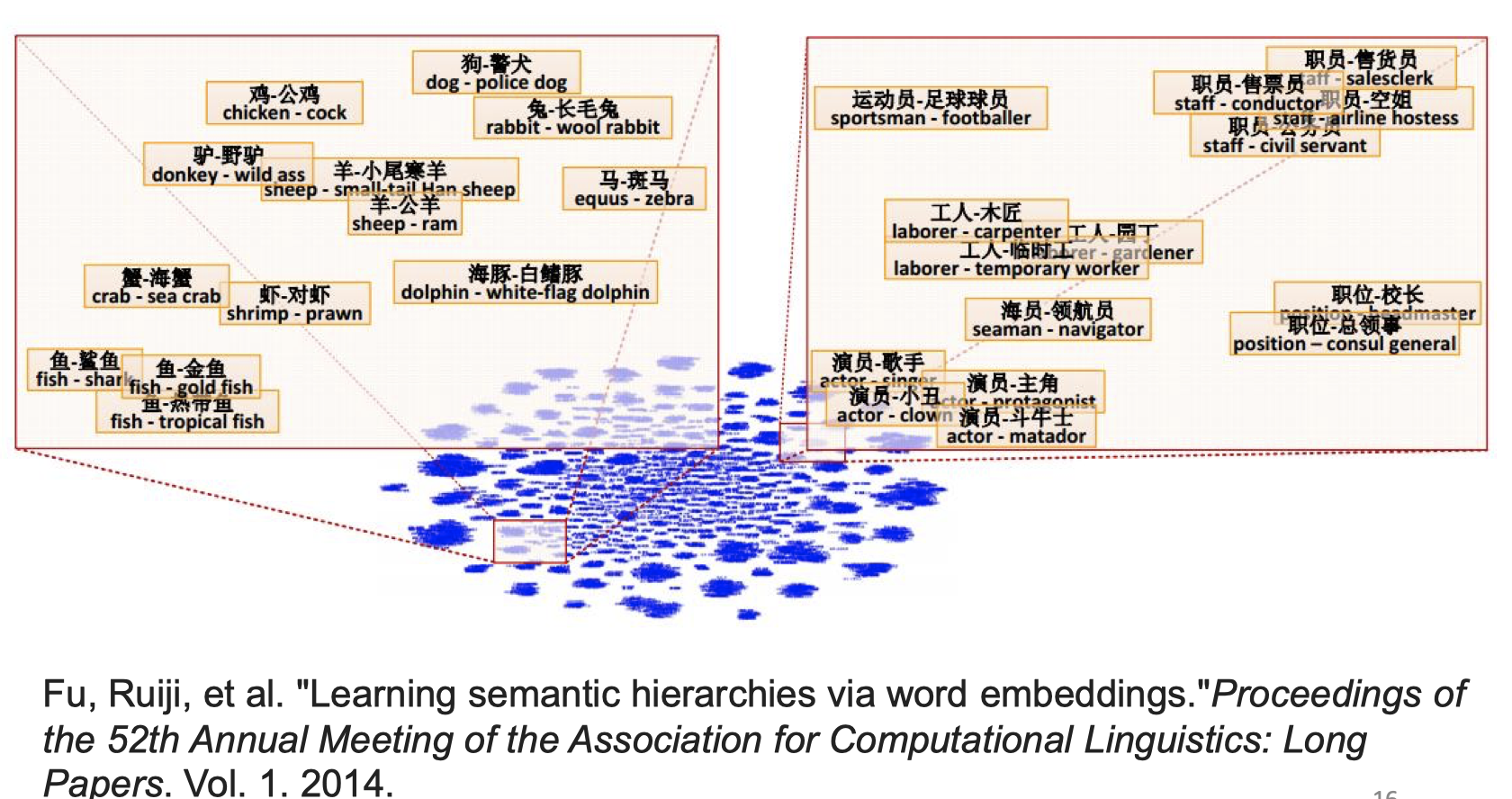• 向量之间表示的语义特性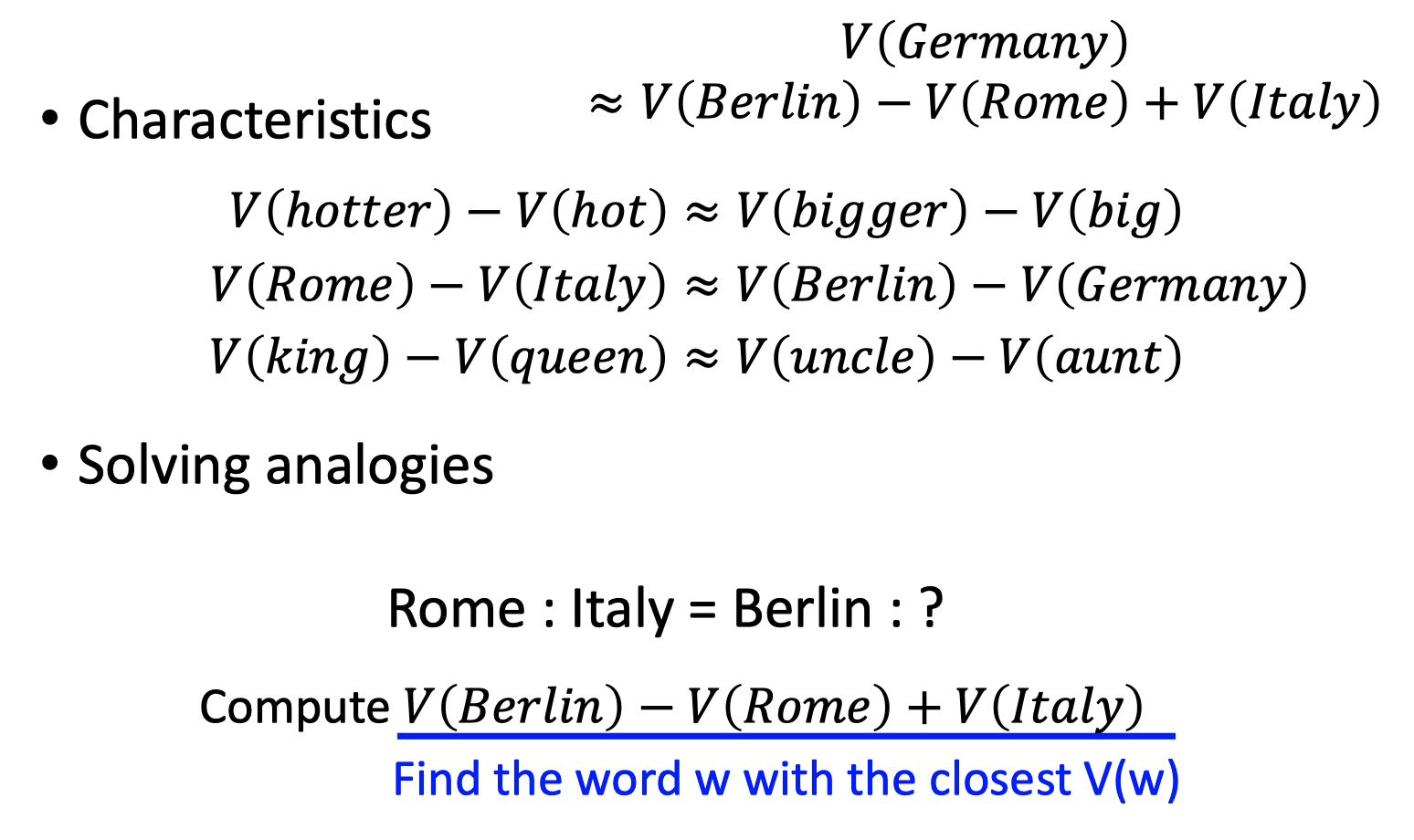## 其他应用

### Multi-lingual Embedding：实现翻译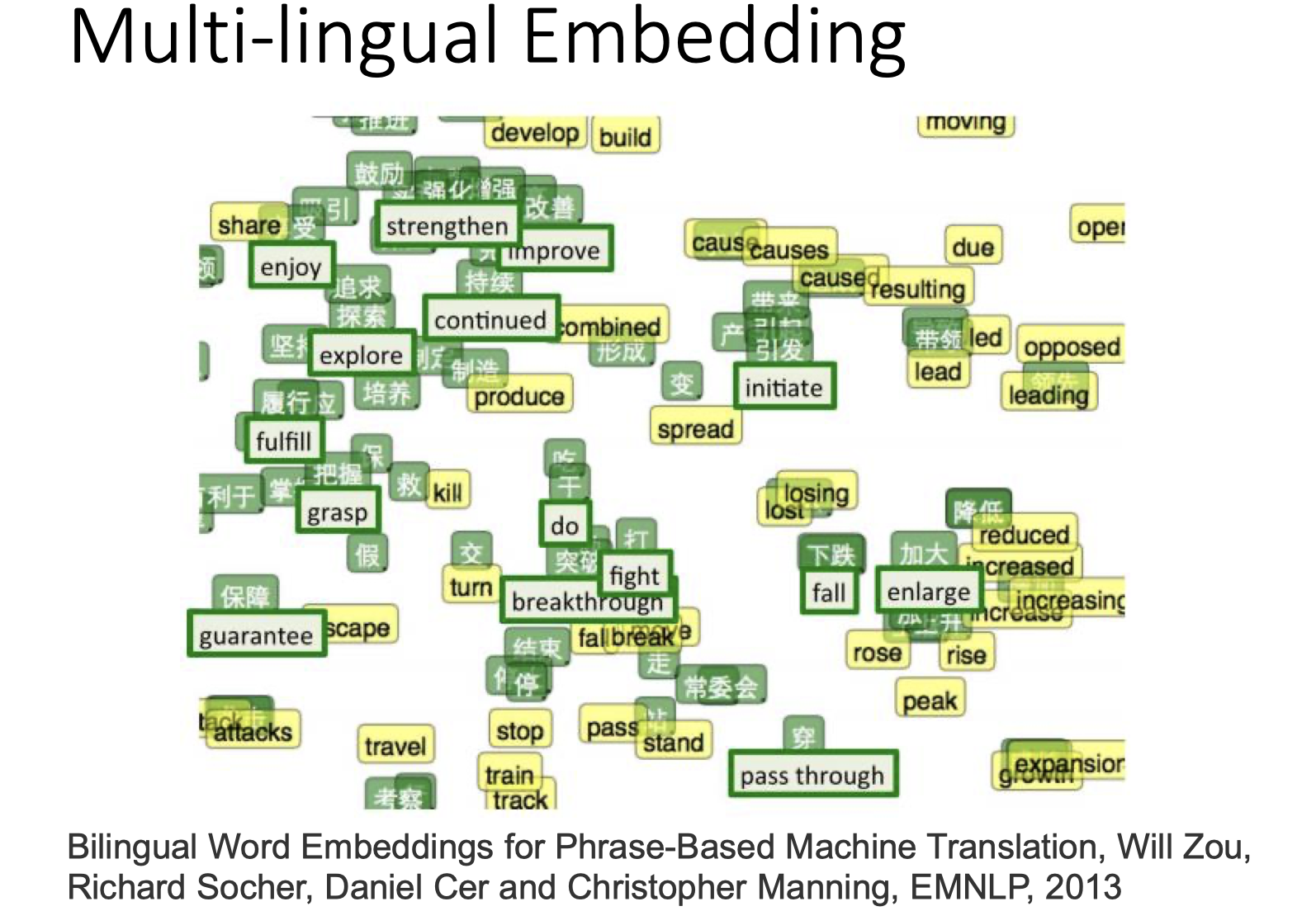### Multi-domain Embedding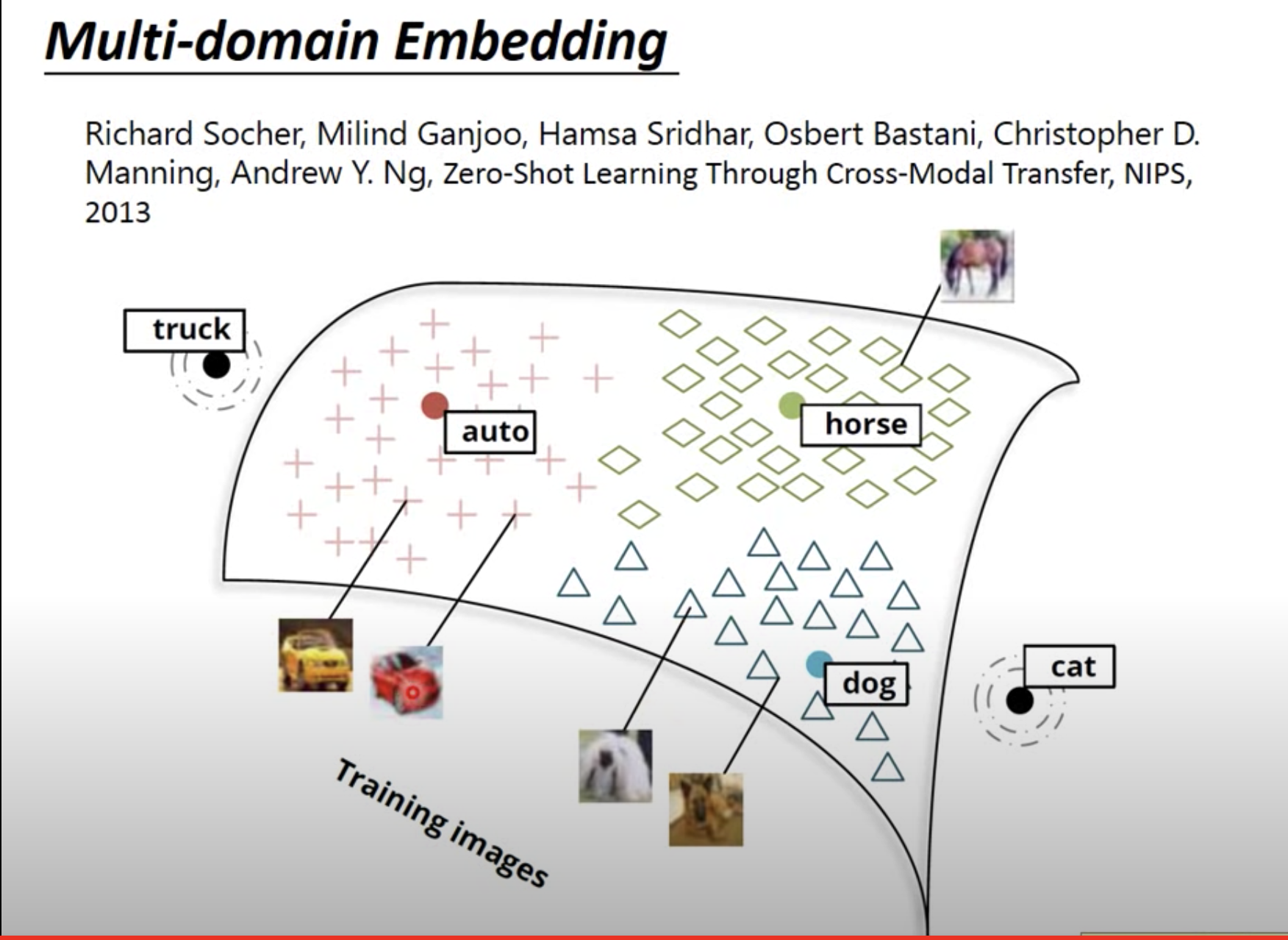### Document Embedding：将文件表示为一个向量

• Bag of Word:

用Bag-of-word的方式编码文件，再实现semantic embedding。得到的文件表示向量可以表示文件的语义主题。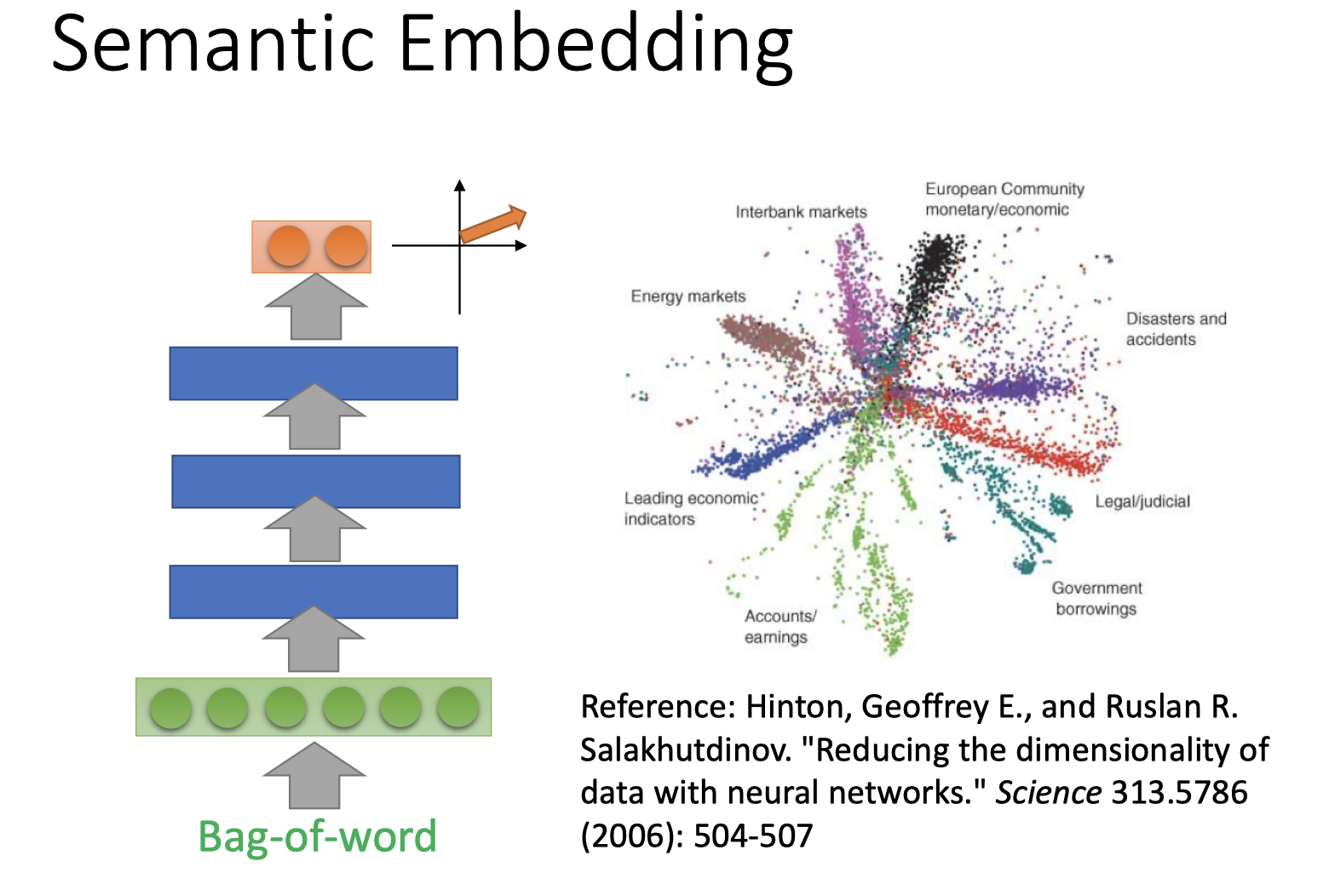• Beyond Bag of Word:

句子中单词的顺序也很大程度影响句子的语义。

因此，下图的两句话有相同的bag-of-word，但表达的含义完全相反。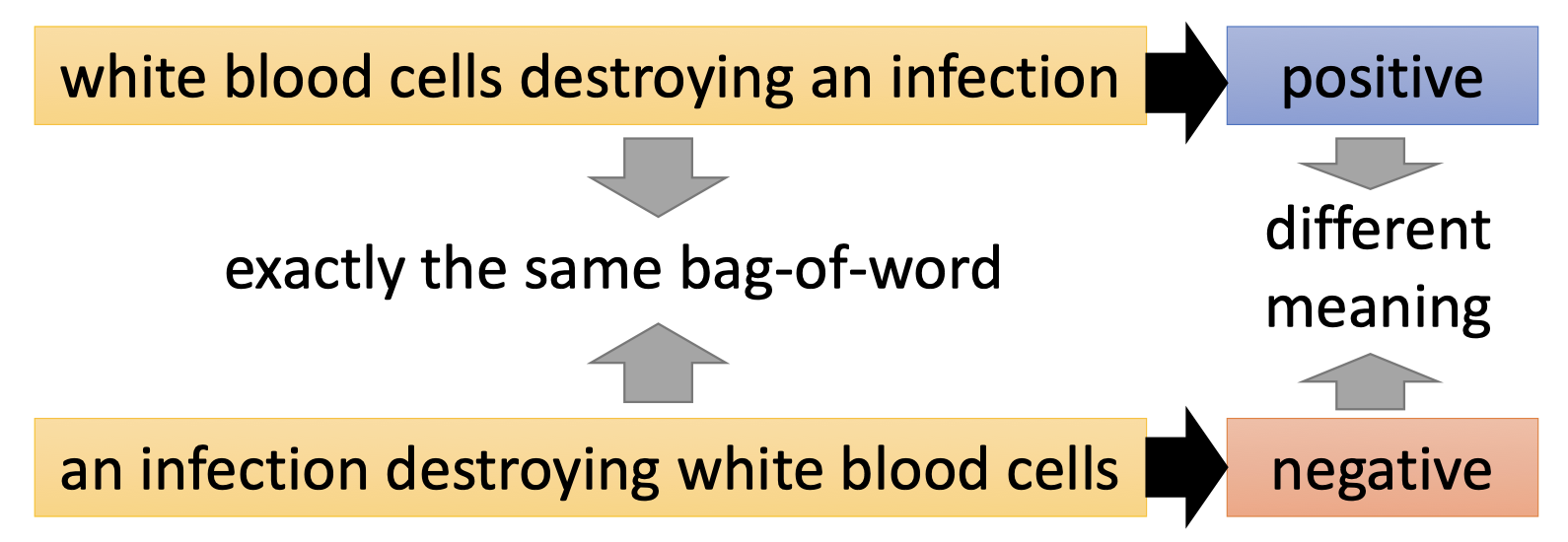关于beyond bag of word的相关工作参考reference 2.

# Reference

1. beyond bag of word: